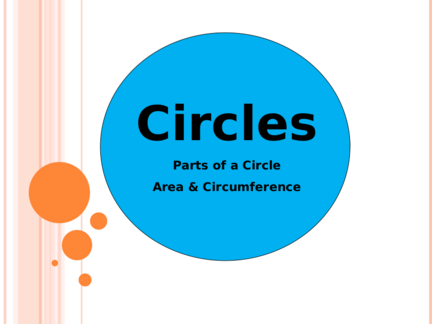# Circumference and Area of CircleContributed by:Circumference of the circle or perimeter of the circle is the measurement of the boundary of the circle. The area of a circle is π multiplied by the square of the radius. The area of a circle(A) when the radius 'r' is given is πr^2. π is approx 3.14 or 22/7.
1. Parts of a Circle
Area & Circumference
2.
3. RADIUS = ½ OF DIAMETER
 If the radius is 5 cm, what is the diameter? 10
 If the diameter is 32 ft, what is the radius? cm
16 ft
58 cm 78 cm
 What is the  What is the
cm cm
4. CIRCUMFERENCE OF A CIRCLE
 Formula is C = 2r (2 x Pi x Radius)
or
C = d (Pi x Diameter)
 Remember… = 3.14 (approximately)
*You can use either formula
depending on what they give you in
the original problem
5. The circumference of a circle
Use π = 3.14 to find the circumference of the following circles:
C = πd 9m C = 2πr
4 cm
= 3.14 × 4 = 2 × 3.14 × 9
= 12.56 cm = 56.52 m
C = πd C = 2πr
58 cm
23 mm = 3.14 × 23 = 2 × 3.14 × 58
= 72.22 mm = 364.24 cm
6. AREA OF A CIRCLE
 Formula is A = r2 (Pi x Radius Squared)
7. The area of a circle
Use π = 3.14 to find the area of the following circles:
2 cm A = πr2 A = πr2
10 m
= 3.14 × 2 2
= 3.14 × 52
= 12.56 cm2 = 78.5 m2
A = πr2 A = πr2
78 cm
23 mm = 3.14 × 232 = 3.14 × 392
= 1661.06 mm2 = 4775.94 cm2
8. Finding the Radius or Diameter
• Circumference • Area
C = 5 in. A = 8.9 m2
Calculate the diameter… Calculate the radius…